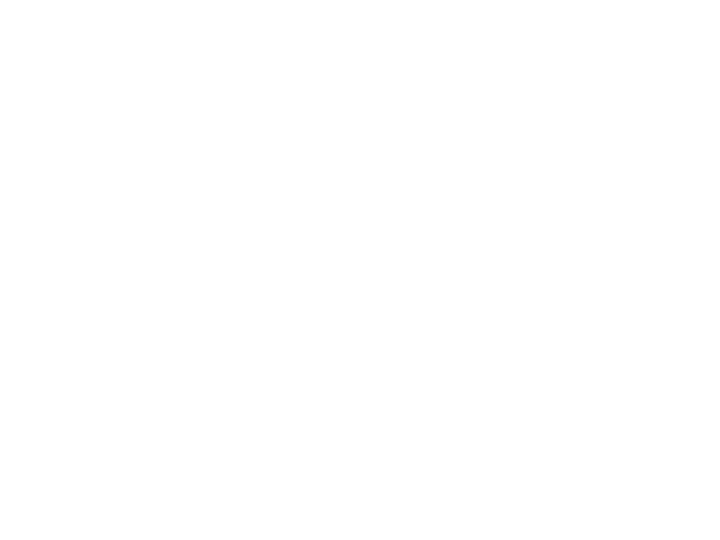Скачать презентацию Finding Percent of a Number Using Fractions Using

3faf4d93fe6f8bf21ed91b6d6034debb.ppt

• Количество слайдов: 19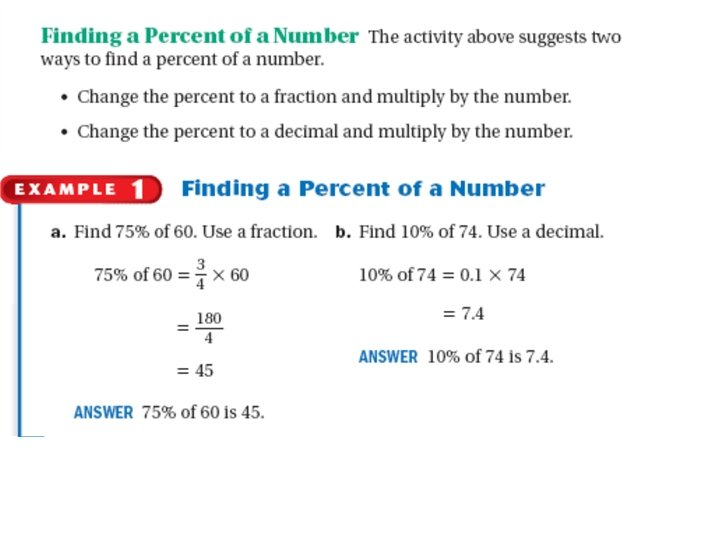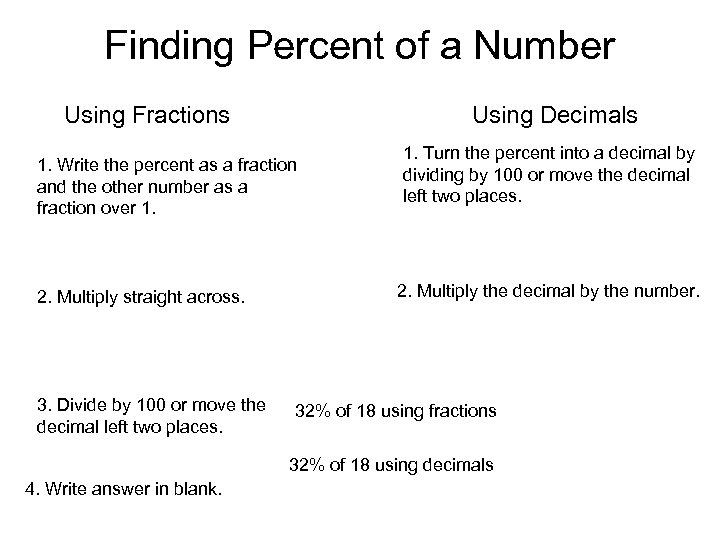Finding Percent of a Number Using Fractions Using Decimals 1. Write the percent as a fraction and the other number as a fraction over 1. 2. Multiply straight across. 3. Divide by 100 or move the decimal left two places. 1. Turn the percent into a decimal by dividing by 100 or move the decimal left two places. 2. Multiply the decimal by the number. 32% of 18 using fractions 32% of 18 using decimals 4. Write answer in blank.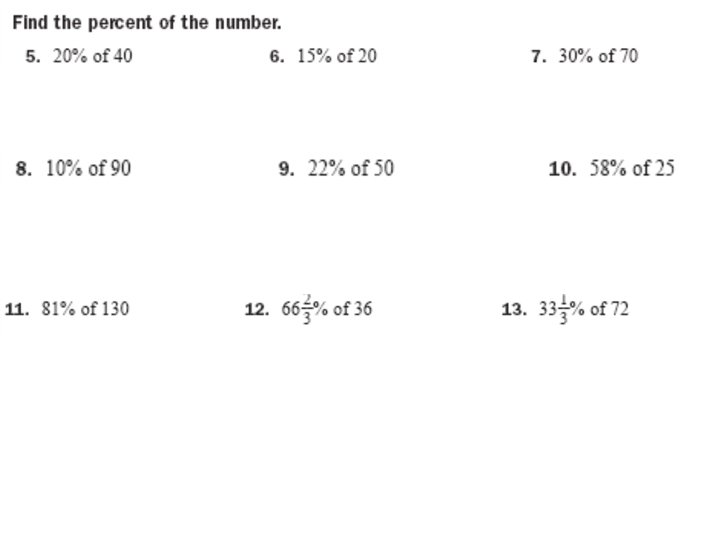Discount: The amount of Money you save. Sale Price: The final cost of the item after you subtract the discount.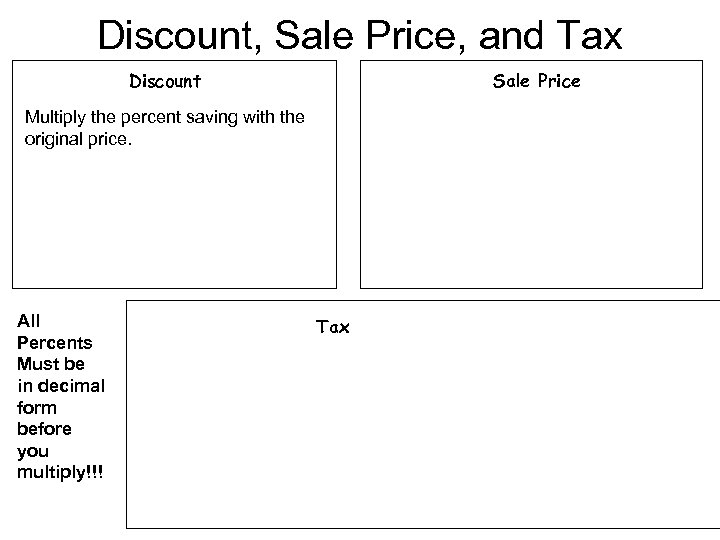Discount, Sale Price, and Tax Discount Sale Price Multiply the percent saving with the original price. All Percents Must be in decimal form before you multiply!!! Tax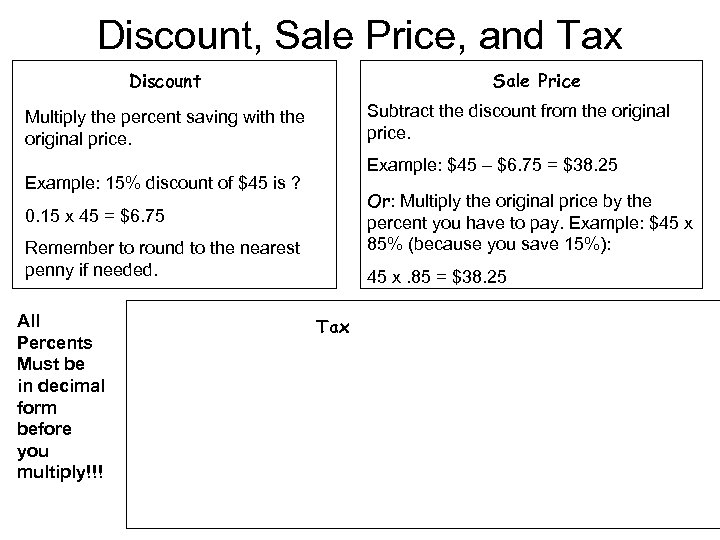Discount, Sale Price, and Tax Discount Sale Price Subtract the discount from the original price. Multiply the percent saving with the original price. Example: \$45 – \$6. 75 = \$38. 25 Example: 15% discount of \$45 is ? Or: Multiply the original price by the percent you have to pay. Example: \$45 x 85% (because you save 15%): 0. 15 x 45 = \$6. 75 Remember to round to the nearest penny if needed. All Percents Must be in decimal form before you multiply!!! 45 x. 85 = \$38. 25 Tax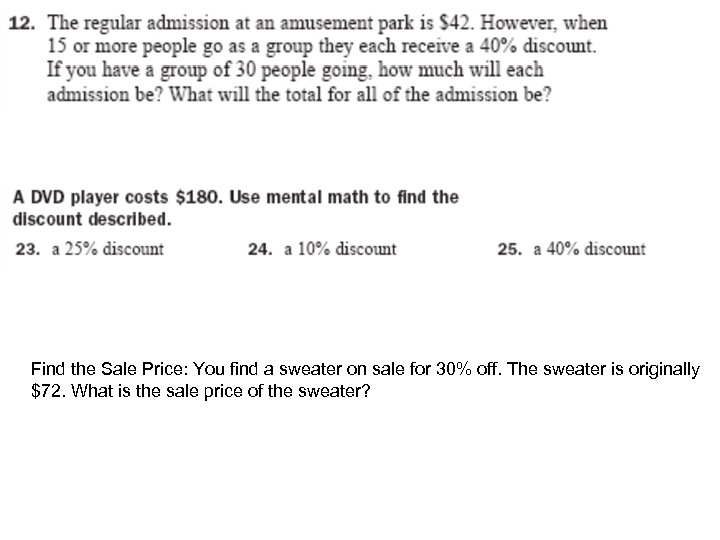Find the Sale Price: You find a sweater on sale for 30% off. The sweater is originally \$72. What is the sale price of the sweater?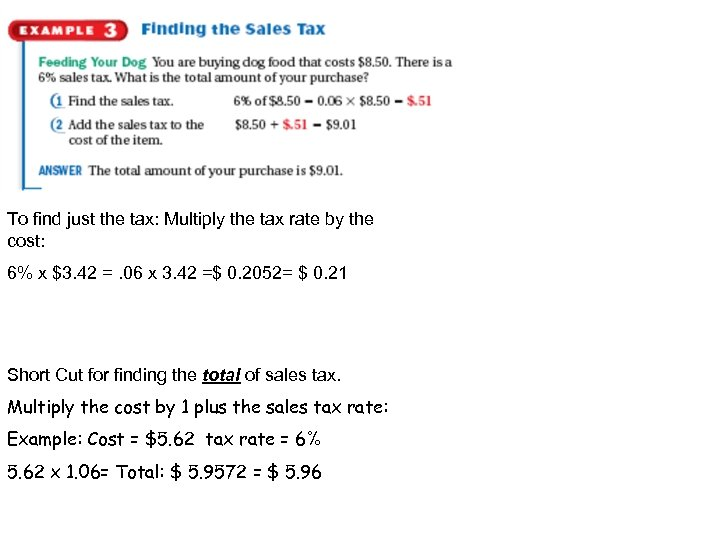To find just the tax: Multiply the tax rate by the cost: 6% x \$3. 42 =. 06 x 3. 42 =\$ 0. 2052= \$ 0. 21 Short Cut for finding the total of sales tax. Multiply the cost by 1 plus the sales tax rate: Example: Cost = \$5. 62 tax rate = 6% 5. 62 x 1. 06= Total: \$ 5. 9572 = \$ 5. 96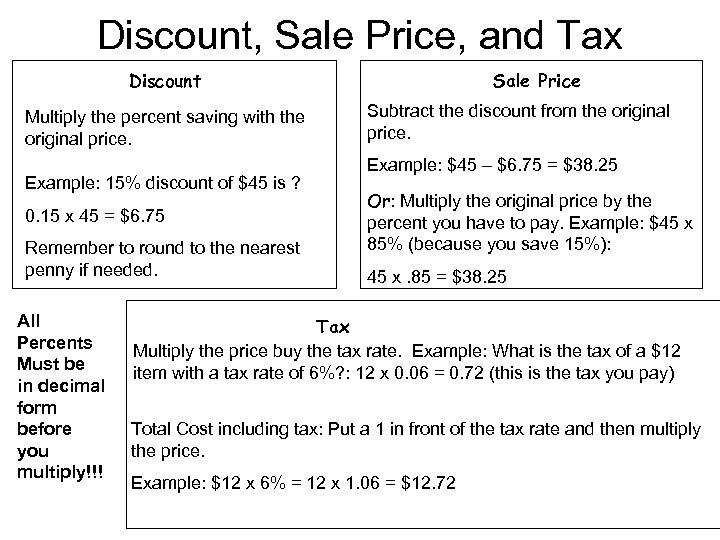Discount, Sale Price, and Tax Discount Multiply the percent saving with the original price. Example: 15% discount of \$45 is ? 0. 15 x 45 = \$6. 75 Remember to round to the nearest penny if needed. All Percents Must be in decimal form before you multiply!!! Sale Price Subtract the discount from the original price. Example: \$45 – \$6. 75 = \$38. 25 Or: Multiply the original price by the percent you have to pay. Example: \$45 x 85% (because you save 15%): 45 x. 85 = \$38. 25 Tax Multiply the price buy the tax rate. Example: What is the tax of a \$12 item with a tax rate of 6%? : 12 x 0. 06 = 0. 72 (this is the tax you pay) Total Cost including tax: Put a 1 in front of the tax rate and then multiply the price. Example: \$12 x 6% = 12 x 1. 06 = \$12. 72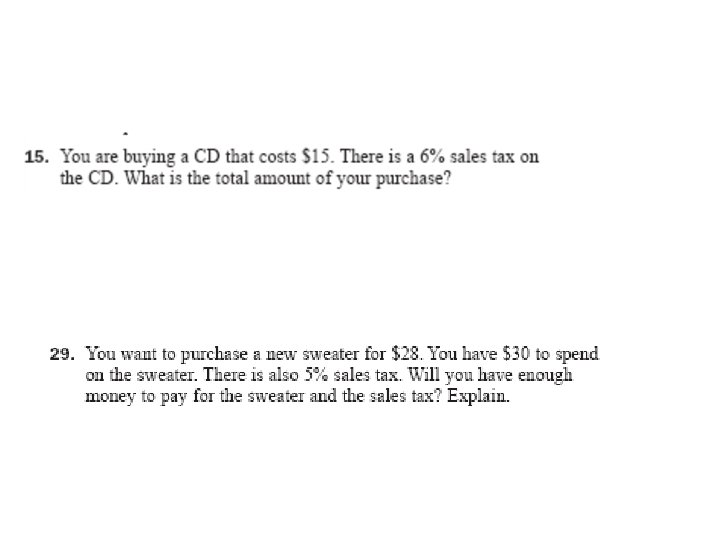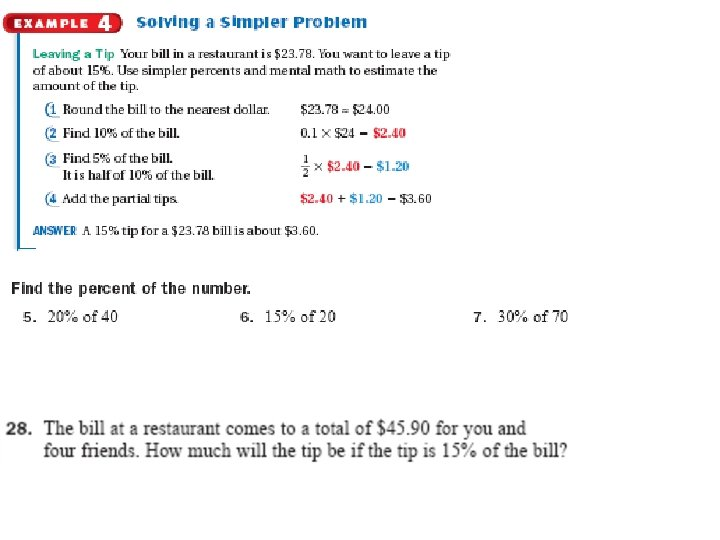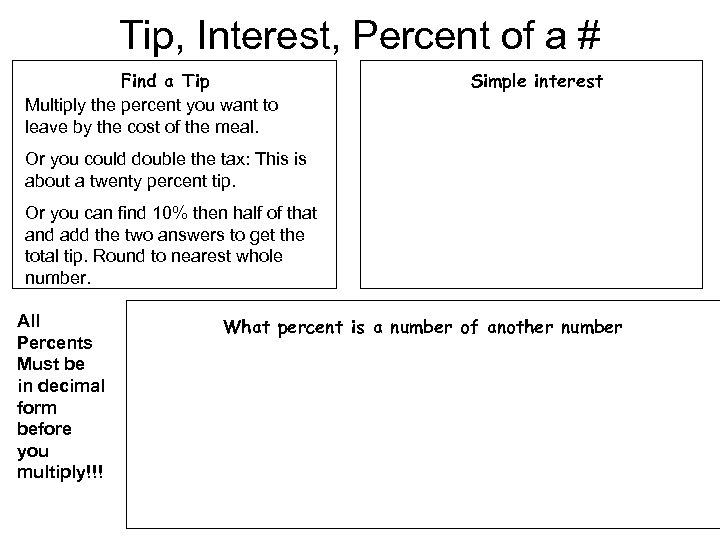Tip, Interest, Percent of a # Find a Tip Multiply the percent you want to leave by the cost of the meal. Simple interest Or you could double the tax: This is about a twenty percent tip. Or you can find 10% then half of that and add the two answers to get the total tip. Round to nearest whole number. All Percents Must be in decimal form before you multiply!!! What percent is a number of another number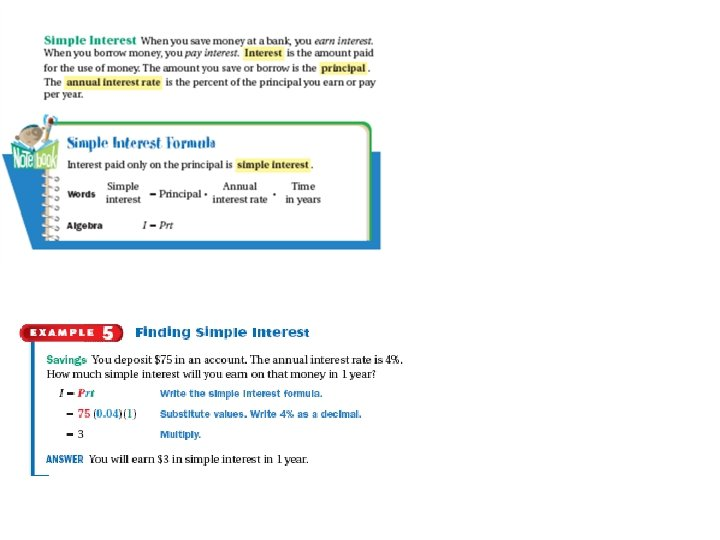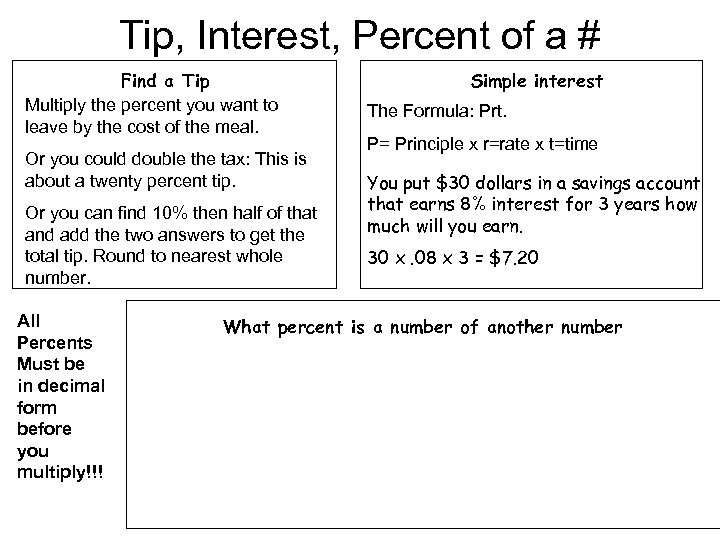Tip, Interest, Percent of a # Find a Tip Multiply the percent you want to leave by the cost of the meal. Or you could double the tax: This is about a twenty percent tip. Or you can find 10% then half of that and add the two answers to get the total tip. Round to nearest whole number. All Percents Must be in decimal form before you multiply!!! Simple interest The Formula: Prt. P= Principle x r=rate x t=time You put \$30 dollars in a savings account that earns 8% interest for 3 years how much will you earn. 30 x. 08 x 3 = \$7. 20 What percent is a number of another number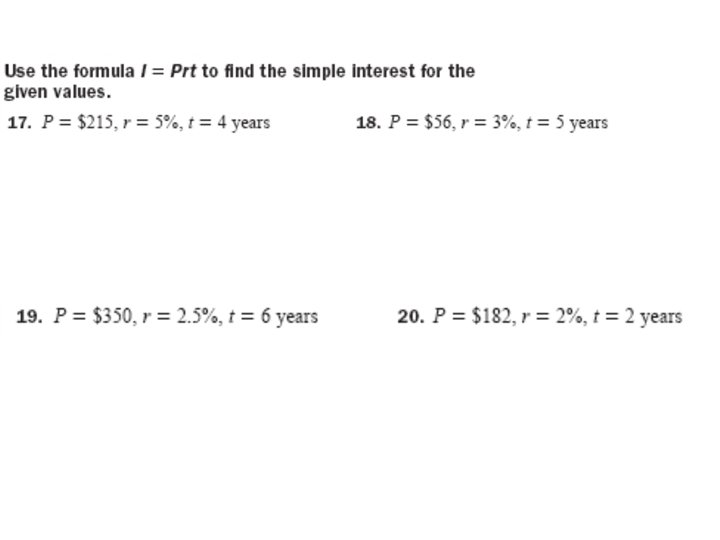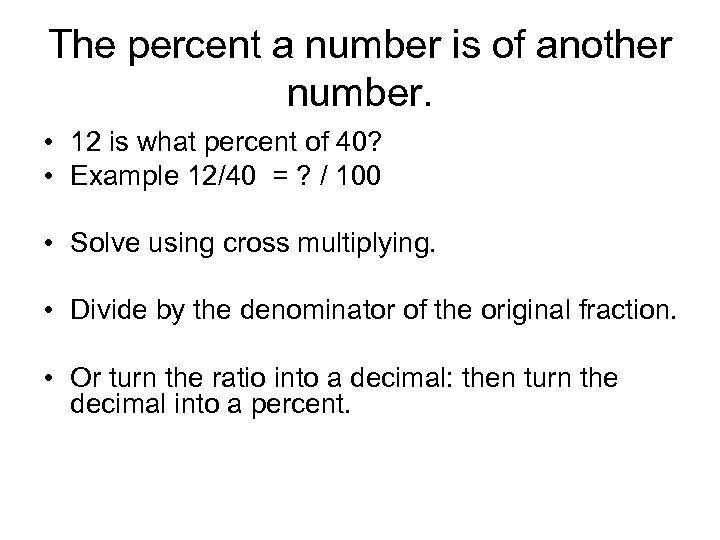The percent a number is of another number. • 12 is what percent of 40? • Example 12/40 = ? / 100 • Solve using cross multiplying. • Divide by the denominator of the original fraction. • Or turn the ratio into a decimal: then turn the decimal into a percent.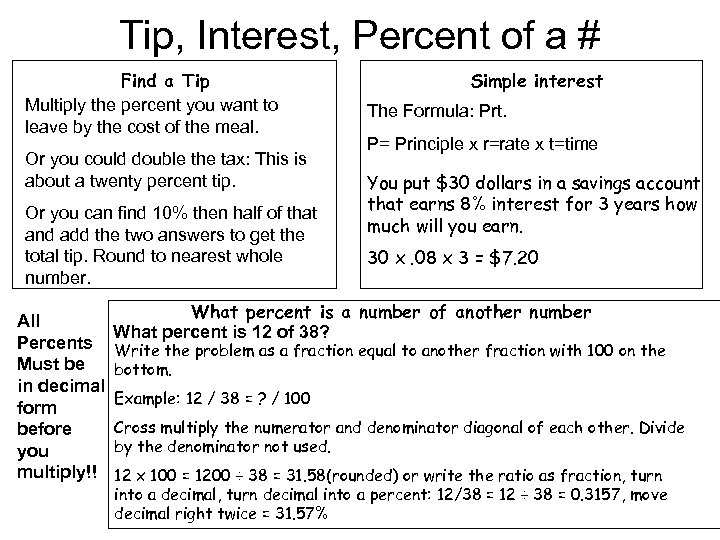Tip, Interest, Percent of a # Find a Tip Multiply the percent you want to leave by the cost of the meal. Or you could double the tax: This is about a twenty percent tip. Or you can find 10% then half of that and add the two answers to get the total tip. Round to nearest whole number. All Percents Must be in decimal form before you multiply!! Simple interest The Formula: Prt. P= Principle x r=rate x t=time You put \$30 dollars in a savings account that earns 8% interest for 3 years how much will you earn. 30 x. 08 x 3 = \$7. 20 What percent is a number of another number What percent is 12 of 38? Write the problem as a fraction equal to another fraction with 100 on the bottom. Example: 12 / 38 = ? / 100 Cross multiply the numerator and denominator diagonal of each other. Divide by the denominator not used. 12 x 100 = 1200 ÷ 38 = 31. 58(rounded) or write the ratio as fraction, turn into a decimal, turn decimal into a percent: 12/38 = 12 ÷ 38 = 0. 3157, move decimal right twice = 31. 57%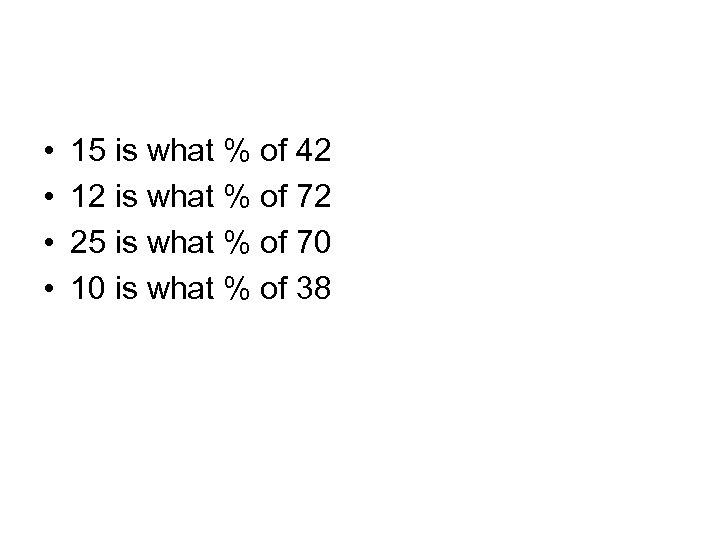• • 15 is what % of 42 12 is what % of 72 25 is what % of 70 10 is what % of 38